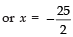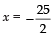Courses

# Value-Based Questions: Polynomials Class 10 Notes | EduRev

## Class 10 : Value-Based Questions: Polynomials Class 10 Notes | EduRev

The document Value-Based Questions: Polynomials Class 10 Notes | EduRev is a part of the Class 10 Course Mathematics (Maths) Class 10.
All you need of Class 10 at this link: Class 10

Ques 1: A charity trust decides to build a prayer hall having a carpet area of 300 square metres with its length one metre more than twice its breadth.
(i) Find the length and the breadth of the hall.
(ii) Which mathematical concept is used in the above problem?
(iii) By building a prayer-hall, which value is depicted by the trust?
Sol:
(i) Let the breadth of the hall = x metres
∴ Length of the hall
= 2 [Breadth of the hall] + 1 metre
= (2x + 1) metre
∴ Carpet area of the hall
= Length × Breadth
= (2x + 1) × x
= 2x2 + x
But the carpet area of the hall = 300 sq.
metre
∴ 2x2 + x = 300
⇒ 2x2 + x – 300 = 0
⇒ 2x2 + 25x – 24x – 300 = 0
⇒ x(2x + 25) –12(2x + 25) = 0
⇒ (x – 12) (2x + 25) = 0
⇒ x – 12 = 0
or 2x + 25 = 0
⇒x = 12Butis not required as the length
or breadth cannot be negative.
∴ x = 12 m and 2x + 1 = (2 × 12) + 1 = 25 m
∴ Breadth of the hall = 12 m
Length of the hall = 25 m
(ii) Quadratic equations
(iii) Enhancing prayer and God fearing feelings.

Offer running on EduRev: Apply code STAYHOME200 to get INR 200 off on our premium plan EduRev Infinity!

## Mathematics (Maths) Class 10

62 videos|363 docs|103 tests

,

,

,

,

,

,

,

,

,

,

,

,

,

,

,

,

,

,

,

,

,

;